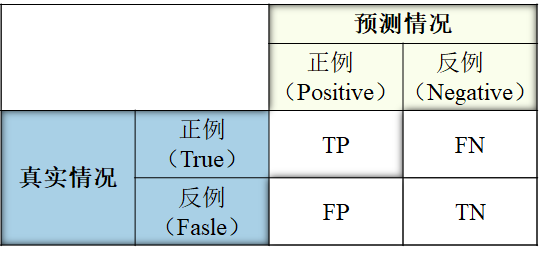Li Shen Blog

Stay hungry, stay foolish.

## 一、线性回归的决定系数$R^2$（也称为判定系数，拟合优度）

$y$为待拟合数值，均值为$\bar y$，拟合值为$\hat{y}$，记：

$R^2 = {SSR \over SST} = {{\sum_{i=1}^{n} (\hat y_i - \bar y)^2} \over {\sum_{i=1}^{n} (y_i - \bar y)^2}} = 1 - {SSE \over SST}$

Python中可直接调用score()方法来计算决定系数$R^2$

score(self, x_test, y_test, sample_weight=None)

## 二、分类问题中的评判标准$accuracy = {{TP + TN} \over {TP + TN + FP +FN}}$

$precision = {TP \over {TP + FP}}$

$recall = {TP \over {TP + FN}}$

$F_1 = 2 \cdot {{precision \cdot recall} \over {precision + recall}}$

P-R曲线ROC空间将假正例率（False Positive Rate, 简称FPR）定义为 X轴，真正例率（True Positive Rate, 简称TPR）定义为 Y 轴。这两个值由上面四个值计算得到,公式如下:
TPR：在所有实际为正例的样本中，被正确地判断为正例之比率。

$TPR = {TP \over {TP+FN}}$

FPR：在所有实际为反例的样本中，被错误地判断为正例之比率。

$FPR = {FP \over {FP+TN}}$AUC(Area Under ROC Curve)

auc其实就是ROC曲线围成的面积！！(a)和(b)展示的是分类其在原始测试集(正负样本分布平衡)的结果，(c)(d)是将测试集中负样本的数量增加到原来的10倍后，分类器的结果，可以明显的看出，ROC曲线基本保持原貌，而Precision-Recall曲线变化较大。

————————————————